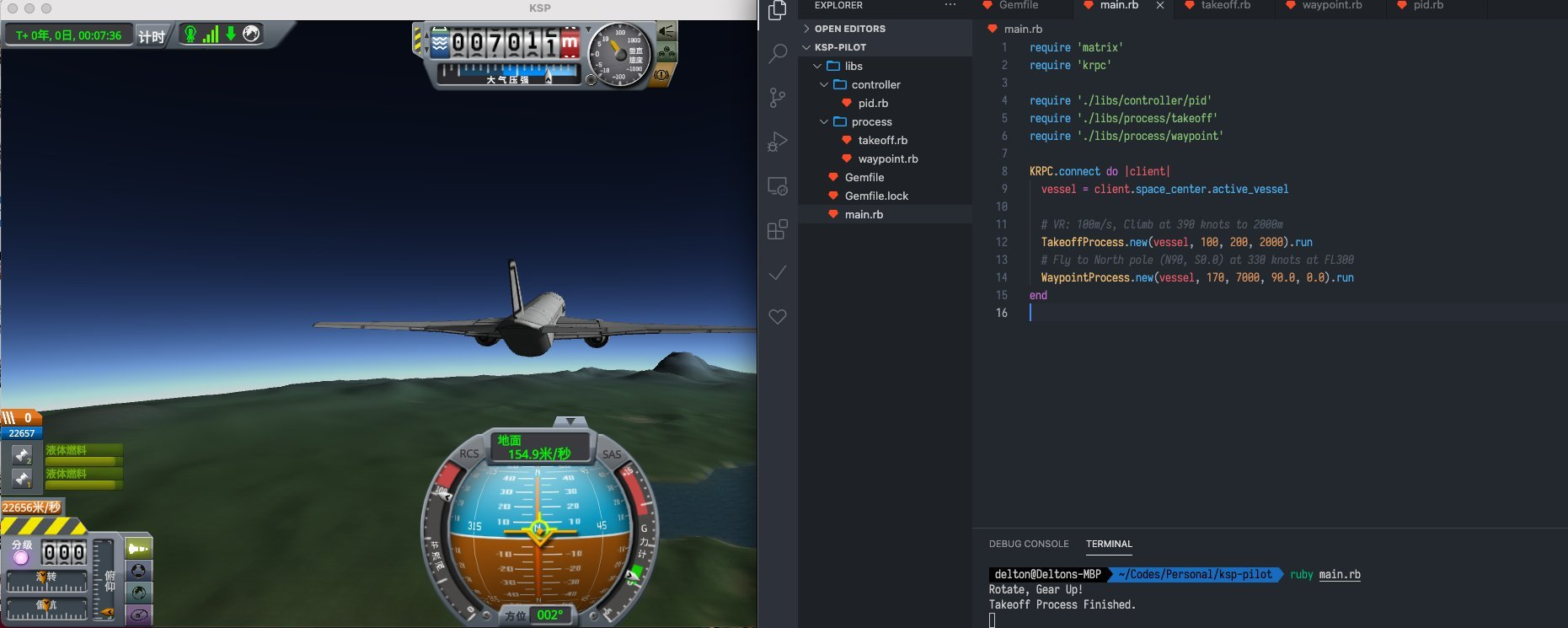# Ruby 用 Ruby 实现飞机自动驾驶仪

dsh0416 · 2021年01月08日 · 最后由 jianglanlan8065 回复于 2021年02月09日 · 1669 次阅读

## krpc

krpc 是一个砍巴拉太空计划（Kerbel Space Program）中的插件。可以通过 RPC 来控制游戏。同时有第三方的 Ruby 客户端：krpc-rb。和真实飞机不同的是，在砍巴拉游戏中驾驶飞机是非常痛苦的，在没有插件辅助的情况下，你看不到具体的 GPS 坐标，很多时候看到的都是和飞机驾驶无关的轨道参数，而一些关键的控制参数缺很难获取。同时游戏中也没有自动驾驶仪。特别在航天飞机降落的控制非常难，虽然手动降落不会太有问题，游戏对于重着陆的容忍度很高，但是要控制飞机飞往机场的过程漫长而痛苦。于是我们试试看利用 krpc 来实现正常商用飞机都有的自动驾驶仪的功能。

## PID 控制

PID 是自动化控制中最基础也是最常用的控制算法。

class PIDController
def initialize(kp, ki, kd, clip_min=0.0, clip_max=1.0)
@prev_err = 0.0
@integral = 0.0
@kp = kp
@ki = ki
@kd = kd
@clip_min = clip_min
@clip_max = clip_max

@last_frame = 0.0
end

def trigger(goal, measured)
trigger_err(goal - measured)
end

def trigger_err(err)
current_frame = Time.now.to_f
dt = current_frame - @last_frame
if dt > 1.0
@last_frame = current_frame
return 0.0
end

@integral = @integral + err * dt

@integral = @clip_min if @integral < @clip_min
@integral = @clip_max if @integral > @clip_max

d = (err - @prev_err) / dt
res = @kp * err + @ki * @integral + @kd * d
@prev_err = err
@last_frame = current_frame

return @clip_min if res < @clip_min
return @clip_max if res > @clip_max
res
end
end



## 起飞控制

class TakeoffProcess
def initialize(vessel, vr, velocity, height)
@vessel = vessel
@control = vessel.control

@vr = vr
@velocity = velocity
@height = height

@throttle_controller = PIDController.new(0.1, 0.01, 0.2)
@pitch_controller = PIDController.new(0.05, 0.05, 0.1, -1.0, 1.0)
end

def run
@control.brakes = false
@control.sas = true
loop do
orbit = @vessel.flight(@vessel.orbit.body.reference_frame)
surface = @vessel.flight(@vessel.surface_reference_frame)
break unless orbit.speed < @vr
throttle = @throttle_controller.trigger(@velocity, orbit.speed)
@control.throttle = throttle
end

# Rotate
puts "Rotate, Gear Up!"
@control.gear = false

loop do
orbit = @vessel.flight(@vessel.orbit.body.reference_frame)
surface = @vessel.flight(@vessel.surface_reference_frame)
break unless orbit.mean_altitude < @height - 100
throttle = @throttle_controller.trigger(@velocity, orbit.speed)
@control.throttle = throttle

pitch = @pitch_controller.trigger(10, surface.pitch)
@control.pitch = pitch
end

puts "Takeoff Process Finished."
end
end



## 方向角计算

delta_phi = Math.log(Math.tan((@latitude / 180 * Math::PI) / 2 + Math::PI / 4) / Math.tan((orbit.latitude / 180 * Math::PI) / 2 + Math::PI / 4))
delta_lon =  (@longitude - orbit.longitude)  / 180 * Math::PI
theta = Math.atan2(delta_lon, delta_phi)
target_heading = theta * 180 / Math::PI


## 偏航控制

1. 控制俯仰来控制升力的原理是，在一定范围内攻角（Angle of Attack）和升力系数成正比。然而当攻角大于某个角度，升力可能会迅速下降（即失速），如果要提高升力应当降低俯仰，而不是继续抬升俯仰。
2. 当滚转角度越来越大后，副翼的升力面会逐渐减小到无论如何提高节流阀大小或调整俯仰都无法维持的情况（即失速），应当将侧倾角度（bank angle）控制在合理范围内。

class WaypointProcess
def initialize(vessel, velocity, height, latitude, longitude)
@vessel = vessel
@control = vessel.control

@velocity = velocity
@height = height
@longitude = longitude
@latitude = latitude

@throttle_controller = PIDController.new(0.1, 0.01, 0.2)
@pitch_controller = PIDController.new(0.05, 0.05, 0.1, -1.0, 1.0)
@roll_controller = PIDController.new(0.0005, 0.01, 0.7, -1.0, 1.0)
end

def run
@control.sas = false
loop do
orbit = @vessel.flight(@vessel.orbit.body.reference_frame)
surface = @vessel.flight(@vessel.surface_reference_frame)
break if (orbit.latitude - @latitude).abs < 1e-4 and (orbit.longitude - @longitude).abs < 1e-4

throttle = @throttle_controller.trigger(@velocity, orbit.speed)
@control.throttle = throttle

pitch = @pitch_controller.trigger(@height, orbit.mean_altitude)
if pitch > 0.0 and surface.pitch > 10
pitch = -0.1
elsif pitch < 0.0 and surface.pitch < -5
pitch = 0.1
end
@control.pitch = pitch

delta_phi = Math.log(Math.tan((@latitude / 180 * Math::PI) / 2 + Math::PI / 4) / Math.tan((orbit.latitude / 180 * Math::PI) / 2 + Math::PI / 4))
delta_lon =  (@longitude - orbit.longitude)  / 180 * Math::PI
theta = Math.atan2(delta_lon, delta_phi)
target_heading = theta * 180 / Math::PI

bank_angle = 25 if delta_heading > 25
bank_angle = -25 if delta_heading < -25

roll = @roll_controller.trigger(bank_angle, surface.roll)
@control.roll = roll
current_roll = surface.roll
end

puts "Waypoint lat: #{@latitude}, lng: #{@longitude} Reached."
end
end



## 测试

require 'matrix'
require 'krpc'

require './libs/controller/pid'
require './libs/process/takeoff'
require './libs/process/waypoint'

KRPC.connect do |client|
vessel = client.space_center.active_vessel

# VR: 100m/s, Climb at 390 knots to 2000m
TakeoffProcess.new(vessel, 100, 200, 2000).run
# Fly to North pole (N90, S0.0) at 330 knots at FL300
WaypointProcess.new(vessel, 170, 7000, 90.0, 0.0).run
end